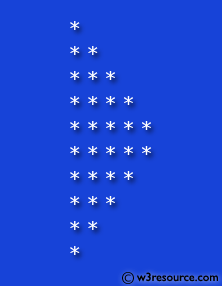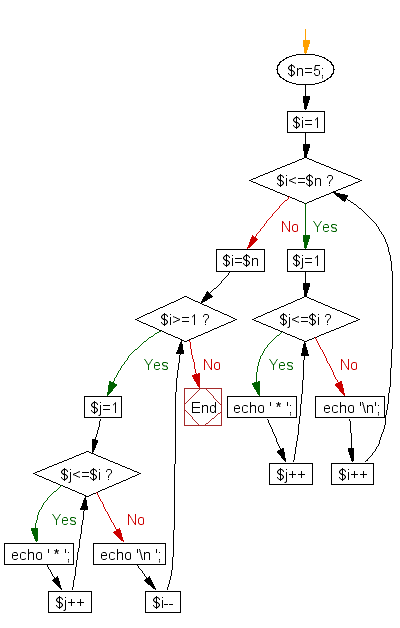﻿ PHP for loops Exercise: Using nested for loop construct a specific pattern - w3resource# PHP for loop Exercises: Using nested for loop, construct a specific pattern

## PHP for loop: Exercise-4 with Solution

Create a script to construct the following pattern, using a nested for loop.

```*
* *
* * *
* * * *
* * * * *
* * * * *
* * * *
* * *
* *
* ```

Pictorial Presentation:Sample Solution:

PHP Code:

``````<?php
\$n=5;
for(\$i=1; \$i<=\$n; \$i++)
{
for(\$j=1; \$j<=\$i; \$j++)
{
echo ' * ';
}
echo '\n';
}
for(\$i=\$n; \$i>=1; \$i--)
{
for(\$j=1; \$j<=\$i; \$j++)
{
echo ' * ';
}
echo '\n ';
}
?>
```
```

Sample Output:

``` *
*  *
*  *  *
*  *  *  *
*  *  *  *  *
*  *  *  *  *
*  *  *  *
*  *  *
*  *
*
```

Flowchart :PHP Code Editor:

Have another way to solve this solution? Contribute your code (and comments) through Disqus.

What is the difficulty level of this exercise?

﻿

## PHP: Tips of the Day

PHP: Merge two arrays while keeping keys instead of reindexing

You can simply 'add' the arrays:

```>> \$a = array(1, 2, 3);
array (
0 => 1,
1 => 2,
2 => 3,
)
>> \$b = array("a" => 1, "b" => 2, "c" => 3)
array (
'a' => 1,
'b' => 2,
'c' => 3,
)
>> \$a + \$b
array (
0 => 1,
1 => 2,
2 => 3,
'a' => 1,
'b' => 2,
'c' => 3,
)
```

Ref : https://bit.ly/3nAP23j